# Mr. R.’s World of Math

## Hypotenuse

### the longest side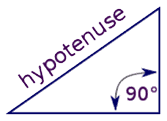Hypotenuse,
Has broken loose!
Going south,
Like a goose…
Now the slide’s,
No fun to ride,
All the children,
Saw and cried!
They liked to slide,
Its longest side!Hypotenuse,
The longest side,
Tried to hide,
But those who know,
Will find him fast,
His game of hiding,
Just won’t last,
He’ll be leaning,
On some trees,
Opposite,
90º
Then we’ll fix,
Old playground slide,
So kids can play,
And glide and ride…

#### The hypotenuse is the longest side of a right triangle.It is the side that is opposite the 90º angle of the triangle.The length of the hypotenuse can be found using the Pythagorean theorem,which states that the squares of the two shorter sides is equal to the square of the hypotenuse.a2 + b2 = c2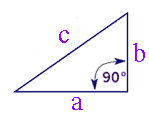#### For example, if the sides of a right triangle are 3, and 4,then 9 + 16 = 25. The length of the third side is the square root of 25 which is 5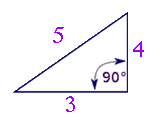RELATED MATH CHALLENGES

If a right triangle has a side of 5 inches, and a hypotenuse of 13 inches, how long is the third side?

A runner runs diagonally across a football field that is 40 yards long and 30 yards wide, how far does the runner run?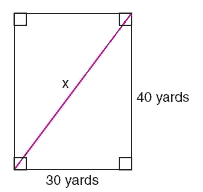A playground slide is 17 feet long, and travels 15 feet along the ground. What is the height of the ladder to the top of the slide? (assume all sides are straight)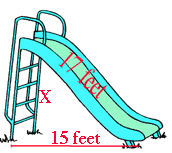RELATED MATH MUSIC VIDEOSRELATED MATH SONGS